Published: 10 October 2014

# Analysis of the pressure wave parameters caused by TNT underwater explosion forced on the hull of minehunter

Grządziela Andrzej1
Szturomski Bogdan3
1, 2, 3Naval Academy in Gdynia, Gdynia, Poland
Corresponding Author:
Grządziela Andrzej
Views 54

#### Abstract

The paper presents the parameters of the pressure wave incident on minehunter hull structure modeled on the simplified vessel hull of Kormoran II project.

In September 2013 Inspectorate of Polish Navy Armament signed a contract to build new ships for combating and setting mines codenamed “Kormoran II”. She has to be adapted to operate in the Polish economic zone, as well as in tactical operations in the Baltic and the North Sea. Basic assumptions tactical – technical shown below :

• Displacement: max: 850 tons;

• Length: 58.00 m;

• Width: 10.30 m;

• Forecastle deck height: 6.40 m;

• The height of the main deck, aft: 4.70 m;

• Draft: 2.60 m;

• Speed: not less than 15 kt;

• Range: not less than 2,500 nautical miles.

Due to the destination of the ship should be examined cases in which the ship may be exposed to the sea mine explosion. It is therefore necessary to distinguish underwater explosion (UNDEX) derived from the enemy charge and the explosion on board in case of a failure while setting up mine warfare. The effects of explosions are difficult to predict. In case of contact explosions which are explosions on board of the ship, it is possible to determine the pressure distribution derived from such an explosion by using the CONWEP procedure which is not described further.

Fig. 1The Kormoran II minehunter designed for the minefield setting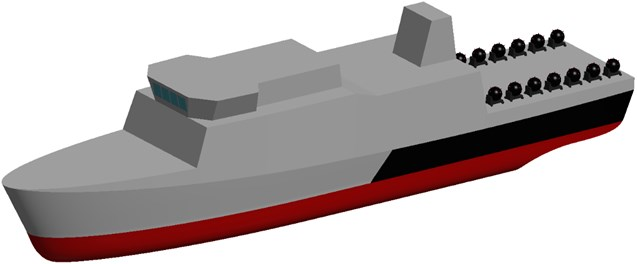Nowaday, many CAE programs uses CONWEP procedure to simple calculations for free explosions in the air. The value of pressure is determined by the program based on the weight of the load and then using TNT equivalents calculated for the respective loads. A similar situation can be applied to underwater explosions. In order to properly calculation of pressure wave incident on the structure shall be familiar with the phenomenon, analytical description of the pressure wave used by the program and simplifying assumptions.

Underwater explosion is a rapid equilibrium violation caused by detonation of explosive in water. During explosion the charge is converted from solid in to has product of volume equal to volume of explosive material, temperature range T 3300 K and pressure p14000 MPa. With so much pressure gas bubble is formed which causes a spherical shock wave that propagates in the medium [3, 4]. The first of the researchers of this phenomenon was the R. H. Cole whose works are the basis for further research. The parameters of the shock wave and the gas bubble parameters are being investigated further. Currently the main of researchers are T. L. Geers, K. S. Hunter and C. K. Park. Most previous publications describes the behavior of gas bubbles, often omitting same shockwave. In this work, omit the description of the bubble in order to focus on the pressure wave from the detonation. This wave in the initial stage moves at a speed of the order of v 5000-8000 m/s. Then, the water molecules act on the water layer adjacent losing speed and travel further the speed of sound in water, which is approximately c0 1500 m/s. Pressure impulse was described by researchers in various ways. It is hard find a universal solution, however, due to the development of measuring instruments in this work decided that for the calculations related to underwater explosion the latest models, ie. model developed by Geers’a and Hunter will be best to use. The following figure shows the profiles described by researchers over the years .

Fig. 2The pressure wave profiles described by researchers over the years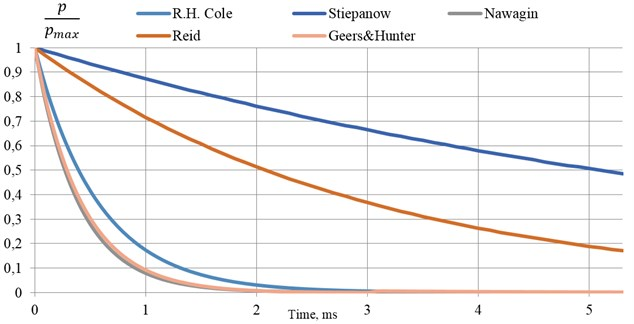Cole formulas shown below. They were determined by the measurement of detonation of 70-136 kg of TNT. Profile of the pressure wave is shown by the following formula:

1
$p\left(t\right)=52.3{\mathrm{}\left(\frac{\sqrt{m}}{r}\right)}^{1,13}\bullet {e}^{-\mathrm{}\frac{t}{\theta }},$
2
$\theta =0.093\sqrt{m}\mathrm{}{\left(\frac{\sqrt{m}}{r}\right)}^{-0,22},$

where: m – charge mass, kg; r – distance of epicenter, m; pmax – peak pressure, MPa; θ – time constant, ms; t – time, ms.

Cole models were presented in 1948. Since that time was corrected by J. S Navagin, W. Stiepanow, and T. L. Geers and K. S. Hunter, the latter formulas are shown below:

3
${p}_{max}={P}_{c}{\left(\frac{{a}_{c}}{r}\right)}^{1+A}\bullet {e}^{-\mathrm{}\frac{t}{\theta }},$
4
$\theta ={v}_{c\mathrm{}}{\left(\frac{{a}_{c}}{r}\right)}^{B},$

where: ac – charge radius, m; Pc= 1.42; vc= 0.992; A= 0.13; B= 0.18.

In the case of explosions of any material other than TNT it is possible to use a TNT equivalent. The equivalent is very difficult to determine because of the multitude of comparative parameters. In practice the heat of explosion, detonation speed and pressure coming from the explosion at a test distance are compared. As the table shows the equivalents determined on the basis of various parameters differ significantly. A good solution is to use average values.

Table 1TNT equivalent for various explosives 

 Explosive Density ρ (g/cm3) Heat of explosion Q (kJ/kg) Pressure p (MPa) Detonation speed D (m/s) TNTe(Q) TNTe(p) TNTe(D) TNT 1.64 5569 19.0 6950 1.00 1.00 1.00 Hexogen 1.8 6334 34.7 8754 1.14 1.83 1.26 Hexotol 90/10 1.61 6232 25.6 7910 1.12 1.35 1.14 Hexotol 80/20 1.6 6150 24.2 7745 1.1 1.27 1.11 Hexotol 70/30 1.59 6070 22.7 7580 1.09 1.19 1.09 Octogen 1.9 6538 39.3 9100 1.17 2.07 1.31 Octol 90/10 1.75 6438 30.3 8320 1.16 1.59 1.2 Octol 80/20 1.71 6342 27.8 8050 1.14 1.46 1.16 Octol 70/30 1.7 6242 26.7 7900 1.12 1.41 1.14 Octol 60/40 1.7 6156 25.3 7680 1.11 1.33 1.11 Pentryt 1.77 6400 33.5 8300 1.15 1.76 1.19 Tetryl 1.68 5920 24.5 7560 1.06 1.29 1.09 PBX-9011 1.77 6168 29.9 8700 1.11 1.57 1.25 HMX/PU 80/20 1.43 5856 19.3 7334 1.05 1.02 1.06 RDX/PU 80/20 1.57 5735 23.4 7778 1.03 1.23 1.12 RDX/PU 70/30 1.38 5436 16.7 6961 0.98 0.88 1.00 NM 1.13 6435 12.5 6523 1.16 0.66 0.94 NGL 1.59 6606 24.6 7580 1.19 1.29 1.09 DATB 1.79 5498 25.9 7520 0.99 1.36 1.08 PETN/PU 90/10 1.65 6406 26.3 7950 1.15 1.38 1.14 PETN/PU 80/20 1.5 6034 21.5 7465 1.08 1.13 1.07 PETN/PU 70/30 1.39 5682 16.5 6957 1.02 0.87 1.00 PEP (85/15) 1.5 6186 21.5 7600 1.11 1.13 1.09 SEMTEX 1.4 6372 19.8 7220 1.14 1.04 1.04 C-4 1.66 6650 25.7 8370 1.19 1.35 1.2 COMP. B 1.72 6000 28.1 8052 1.08 1.48 1.16 LX-17 1.91 4407 31.6 7630 0.79 1.66 1.1 LX-14 1.83 6452 36.3 8958 1.16 1.91 1.29

The task of explosions, should be regarded as an appropriate task described by the equation of motion. Recognition of this phenomenon using the finite element method requires the use of an appropriate equation in the form:

5
$\mathbf{M}\left(\mathbf{U}\right)\stackrel{¨}{\mathbf{U}}+\mathbf{C}\stackrel{˙}{\mathbf{U}}+\mathbf{K}\left(\mathbf{U},\stackrel{˙}{\mathbf{\epsilon }}\right)\mathbf{U}=\mathbf{F}\left(t,R,{m}_{TNT}\right),$

where: K – stiffness matrix; M – matrix of inertia (density matrix); C=αM+βK – damping matrix, where α and β are constant coefficients; U,U˙,U¨ – displacement, velocity and acceleration vector; F – load vector, ε˙ – strain rate vector; t – time; R – distance from epicenter; mTNT – TNT charge mass.

In the task load vector F have been set as a time-varying pressure wave in accordance with Eqs. (1), (2) or (3), (4). Other expressions of Eq. (5) in this work are omitted, because they describe the structure reaction while this work refers only to load.

Polish Navy is currently using 4 types of sea mines. Among other, uses OS mine which contains 110 kg TNT (Source: Laboratory of Underwater Weapons Polish Naval Academy). The paper examined a case in which such a charge detonates around the ship of Kormoran II project. Using CAD programs reflected outer shell of the ship, and then implements it into CAE program in which the relevant calculations were carried out.

Charge was placed at a distance of 9 m below the keel of the ship. According to Eq. (3) the pressure on the front of the shock wave is 25.67 MPa. The pressure calculated by the program was 29 MPa. The difference results from the strong relationship between the size and shape of mesh elements. In the paper  the effect of mesh size and shape on the propagation of the shock wave were analyzed. It was found that the 4 – nodal tetragonal of size of 0.5 m elements are best to use.

Fig. 3Pressure distribution of non-contact TNT explosion under the keel of the ship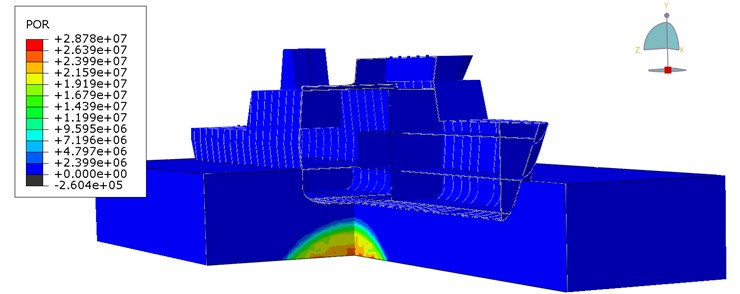a)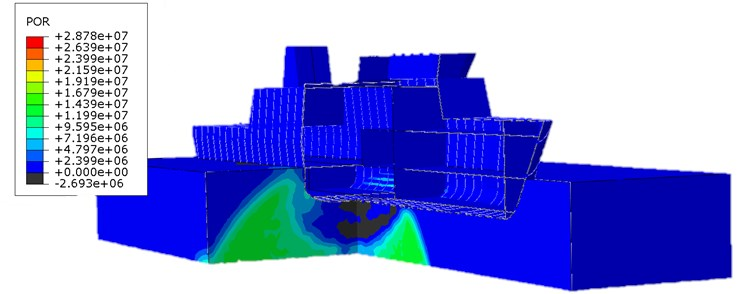b)

Fig. 4Pressure distribution of non-contact TNT explosion under wet surface of the ship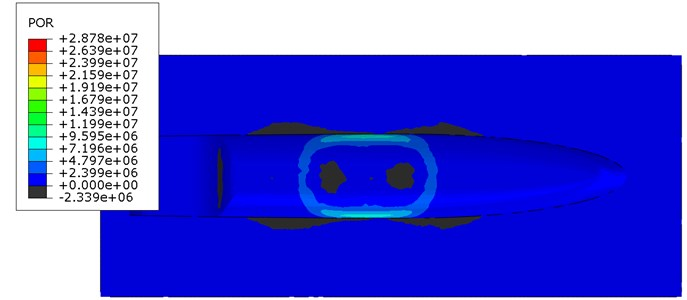The pressure distribution of non-contact mine explosion in the acoustic medium in different time points were shown. The pressure wave reaches the ship’s hull and it is reflected resulting in a negative pressure. The decrease of pressure can lead to cavitation under the keel of the ship. In this case, if the ship is treated as a beam supported continuously, due to cavitation a discontinuity of the support will appear and the ship would be load with the forces of gravity. In this work, this phenomenon is omitted.

Study describes the phenomenon of underwater explosion. The model used in the study is the latest model of the shock wave in this type calculations. Problems that results from the application of the acoustic medium and its response for the given load. Also highlights the FEM equation that describes the dynamics task. The complexity of this task depends on the author and expected results. In case of underwater explosions weight loss, elements self – contact or hardening of the material due to the strain rate can be included. The influence of various parameters on the results will be examined in further publications.